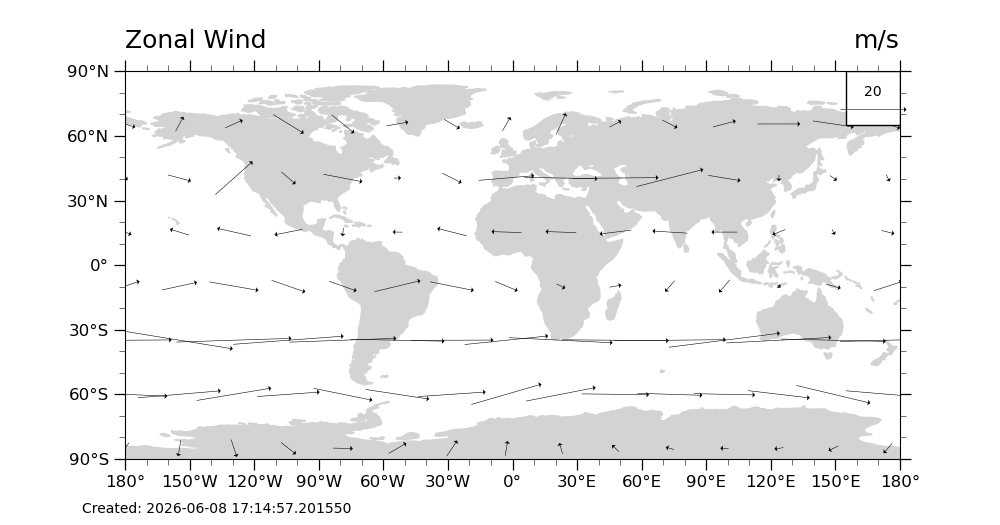# NCL_vector_3.py#

Plot U & V vectors globally

This script illustrates the following concepts:
• Drawing a black-and-white vector plot over a PlateCarree map

• Adding a time stamp to a plot

• Moving the vector reference annotation to the top right of the plot

See following URLs to see the reproduced NCL plot & script:

Import packages:

```import xarray as xr
from matplotlib import pyplot as plt
import cartopy
import cartopy.crs as ccrs
from datetime import datetime

import geocat.datafiles as gdf
import geocat.viz as gv
```

```# Open a netCDF data file using xarray default engine and load the data into xarrays
file_in = xr.open_dataset(gdf.get("netcdf_files/uv300.nc"))

# Extract slices of lon and lat
# Read in data from netCDF file.
# Note that when we extract ``u`` and ``v`` from the file,
# we only read every third latitude and longitude.

ds = file_in.isel(time=1, lon=slice(0, -1, 3), lat=slice(1, -1, 3))
```

Plot:

```# Generate figure (set its size (width, height) in inches)
plt.figure(figsize=(10, 5.25))

# Generate axes using Cartopy projection
ax = plt.axes(projection=ccrs.PlateCarree())
z = gv.set_vector_density(ds, 0.017)

# Draw vector plot
# Notes

# 1. We are using `set_vector_density` on line 47 as a replacement for NCL's vcMinDistanceF
# 2. There is no matplotlib equivalent to "CurlyVector"
Q = plt.quiver(z['lon'],
z['lat'],
z['U'].data,
z['V'].data,
color='black',
zorder=1,
pivot="middle",
width=0.0007,

# Draw legend for vector plot
qk = ax.quiverkey(Q,
167.5,
72.5,
20,
r'20',
labelpos='N',
coordinates='data',
color='black',
zorder=2)

edgecolor='lightgray',
facecolor='lightgray',
zorder=0)

# Draw the key for the quiver plot as a rectangle patch
plt.Rectangle((155, 65),
25,
25,
facecolor='white',
edgecolor='black',
zorder=1))

# Use geocat.viz.util convenience function to set axes tick values
gv.set_axes_limits_and_ticks(ax,
xticks=range(-180, 181, 30),
yticks=range(-90, 91, 30))

# Use geocat.viz.util convenience function to add minor and major tick lines

# Use geocat.viz.util convenience function to make plots look like NCL plots by using latitude, longitude tick labels

# Use geocat.viz.util convenience function to add titles to left and right of the plot axis.
gv.set_titles_and_labels(ax,
lefttitle=ds['U'].long_name,
righttitle=ds['U'].units)```/home/docs/checkouts/readthedocs.org/user_builds/geocat-examples/conda/latest/lib/python3.10/site-packages/geocat/viz/util.py:1419: UserWarning: Plot spacing is alrady greater or equal to 0.017